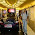## Wednesday, April 13, 2011

### Typeof vs GetType (how to compare types)

Let say I have implemented few classes in following manner

Public Interface IA
Property Id As String
End Interface

Public Class A
Private _id As Integer
Public Overridable Property Id As Integer
Get
Return _id
End Get
Set(ByVal value As Integer)
_id = value
End Set
End Property
End Class

Public Class B
Inherits A
Implements IA

Public Property Id As String Implements IA.Id

Get

End Get
Set(ByVal value As String)

End Set
End Property
End Class

Now I write switch statement to compare type like these ways

Dim a As IA = New B
a.Id = "2"
Dim b As A = New B
b.Id = "2"

Dim t As String = a.GetType.ToString
Select Case t
Case GetType(A).ToString
Console.WriteLine("type is a")
Case GetType(B).ToString
Console.WriteLine("type is b")
End Select

Select Case True
Case TypeOf a Is A
Console.WriteLine("type is a")
Case TypeOf a Is B
Console.WriteLine("type is b")
End Select

Select Case True
Case TypeOf b Is A
Console.WriteLine("type is a")
Case TypeOf b Is B
Console.WriteLine("type is b")
End Select

Now note that the yellow one will print “type is b” but green one will print “type is a”. actually "is" operator traverses the complete hierarchy to match the type so typeof a is “IA”,”A”,”B” all true. But GetType does not return you the type of reference instead actual type since it is calculated on runtime so it will just give you a.GetType = B only.

Now let me share with you some interesting methods from System.Type namespace which works ofcourse on runtime and can be very handy.

Let me first define some dummy interfaces, classes and their relations.

interface IBase
{

}

interface IA : IBase
{

}

class A : IA
{

}

class B : A
{

}

Now let me create few objects out of that

IBase ibase = new B();
IA ia = new B();
A a = new A();
B b = new B();

Now I want to see if type of my object b is derived from type of my object a. There are two methods
1. Type.IsAssignableFrom
2. Type.IsSubclassOf

// x.IsAssignableFrom(y) returns true if:
//   (1) x and y are the same type
//   (2) x and y are in the same inheritance hierarchy
//   (3) y is implemented by x
//   (4) y is a generic type parameter and one of its constraints is x

IsSubclassOf function does just reverse of IsAssignableFrom. So if (typeof)x.IsAssignableFrom(typeof(y)) is true then (typeof)y.IsSubclassOf(typeof)x) will also be true.
Kindly note that the above two functions are valid on concrete types only. I will explain how to get types for interface in a minute, hang on.

let's get some working examples (refer above mentioned classes and objects)

1. ia.GetType().IsAssignableFrom(a.GetType()) will return false since actual type of ia is "B".

2. a.GetType().IsAssignableFrom(ia.GetType()) will return true since object of type of ia ("B") can be assigned to type of a ("A")

3. b.GetType().IsAssignableFrom(a.GetType()) will return false since b is not assignable from a type

Now some examples of IsSubclassOf

1. a.GetType().IsSubclassOf(ia.GetType()) will return false since ia (type "B") is not base class of a (type "A").
2. b.GetType().IsSubclassOf(a.GetType()) will return true since "B" is subclass of "A".

Let's discuss about checking the interface type now

If I want to see how many interfaces are implemented by the type of my object we can use GetInterfaces method

Type[] types = ia.GetType().GetInterfaces();
will give me types = "IBase" and types = "IA"

If I want to see whether a particular type is implemented by my object type, I can use GetInterface method and pass interface name as string parameter.

Type type = ia.GetType().GetInterface("IA");
will return me IA (interface type) since type of ia ("B") implements it.

similarly
Type type = b.GetType().GetInterface("IBase");
will also return me IBase type since my object b has that in its hierarchy.

Now what if I do something like
Type type = ia.GetType().GetInterface("I");
where I is some unrelated interface. you might have guessed it correctly type object will be null and we can tell that my object type never has interface "I" type in its complete hierarchy.

#### 3 comments:

1.typeof() function does not traverse the class hierarchy, actually in above example, results are different due to use of "is" keyword.
b is A returns true if typeof(b) can be cast into A otherwise returns false. Since B is derived from A, that is why
TypeOf a Is A returns true (a is object of B here).

typeof() vs GetType():
typeof() is a static type lookup, essentially it occurs at compile time, so you only get the explicitly named type.
GetType() is a dynamic type lookup, it's a virtual method that gets called at runtime and will give you the exact type even if you are using polymorphism. So it's "slower", theoretically, but it's giving you something you can't get from typeof(T). If you need one or the other for your design, the speed isn't going to be a factor.

2.Thanks. I shall be making the appropriate correction. I am also adding up more useful information for type comparison.

3.